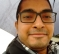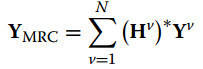## Confusion with Maximal Ratio Combining(MRC) in SIMO OFDMStarted by 6 years ago6 replieslatest reply 6 years ago765 views

My basic question is that in SIMO OFDM system with Maximal Ratio Combining :

Do we need to do equalization after MRC or just send the combined signal for further steps i.e. steps after equalization?

(Apology if I am asking something trivial)

Here are details :

Consider a 1 X V SIMO OFDM system. (802.11g system, FFT size 64, data subcarrier 48).

After FFT on all V RX ports, the channel estimate for the V channels are obtained. The channel estimates are 48 X 1 each.

Using those channel estimates we do MRC combining of V Rx streams according to following equationwhere Yv is complex sample stream 48 X 1 from Vth antenna. Hv is a diagonal matrix 48 x 48 with diagonal elements as channel estimate for Vth antenna. The output of the above equation will be summation of V 48 X 1 matrices i.e a single 48 X 1 matrix.

Now after this, this, does YMRC needs to be equalized ? For example using MMSE or ZF etc ?

If yes, which channel estimate out of V such channel estimates will be used for equalization ? Because for every antenna, there is a channel estimate 48 X 1.

Expression for ZF and MMSE are below :

ZF equalizer:  1/H[i]
MMSE equalizer:  H*[i]/ (H[i].H*[i] + N0)

Where H[i] is the channel coefficient for the ith subcarrier on Vth RX stream and N0 is the noise variance on the Vth RX antenna.

[ - ]If you do the combining first, you will get the SNR improvement of the combiner and will be equalizing on the combined channels.   If you do the equalizing first, you will get the SNR improvement on the equalized channels.   Which one yields the best improvement may depend on the channels and the implementation of the equalizers.

Combining first may reduce complexity if it is cheaper to have one combiner and one equalizer rather than two equalizers and one combiner.

In general, though, or at least in my experience it is often beneficial to combine after equalization.   Again, though, this may be channel and system dependent, especially if complexity is a significant concern.

[ - ]As you said "If you do the combining first, you will get the SNR improvement of the combiner and will be equalizing on the combined channels."

So for the equalization step, which channel estimate will be used because now after combining, I have one signal vector but multiple channel estimate vectors(one from each of the RX path).

[ - ]If you combine first before equalization, you have to train the equalizer on the combined signal, not either of the pre-combined signals.

[ - ]Ohh I see! So let me know if my understanding is correct (thanks much for your time :))Considering the case of 802.11g system where long training sequence(LTS) is used for channel estimation.

Step-1 I use LTS for getting channel estimation(chest) on branch A and B, say Ha and Hb.

Step-2 I do MRC on the two RX streams from A and B using Ha* and Hb* and I get a stronger signal. Using MRC, I combine the entire OFDM frame including LTS and the payload.

Step-3 Now I use the LTS of the combined signal to again estimate the channel say Hmrc

Step-4 I do equalization of the stronger signal using Hmrc

[ - ]